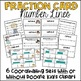# Fraction Number Line Cards & Fraction Model Cards Teacher Tools ~ Over 400 CardsSubject
Resource Type
File Type
PDF (23 MB|144 pages)
Standards
\$3.00
• Product Description
• Standards

This Teacher Tools Set includes over 400 Fraction Number Line and Fraction Models Cards. Perfect for teaching fraction number sense, comparing fractions, equivalent fractions, & benchmark fractions. Designed for multiple grade levels, halves through twelfths included, 6 different coordinating sets. Common Core and TEKS Aligned resource! Use as a teaching tool to introduce, review, and practice fraction number sense.

What’s Included:

✅ 6 Different Coordinating Sets of Fraction Number Line Cards

✅ Provided in 2 Different Themes: With Doodle Kids Clipart and Without

✅ Designed for Multiple Grade Levels (halves through twelfths included)

✅ Bonus Fraction Game Direction Cards

✅ Over 400 Fraction Cards

Ideas for Use in Classroom:

►Lesson Resource: Have a variety of sets available and use as an additional tool when introducing or practicing a fraction skill.

►Guided Math: Use as a teaching tool during guided math and intervention. This resource provides a variety of ways to think about and present fractions.

►Math Centers: Have a variety of sets available to go along with any fraction math station activity. A great addition for any fraction math center activity.

►Fraction Number Sense Games: There are numerous games to play using these fraction cards. Included are direction cards for 4 different games.

FOLLOW ME for New Products, Sales, and Freebies!

❤️ I hope this description helps to clarify! If you have any questions or would like to contact me feel free to email me at solanikcurriculum@gmail.com

Compare two fractions with different numerators and different denominators, e.g., by creating common denominators or numerators, or by comparing to a benchmark fraction such as 1/2. Recognize that comparisons are valid only when the two fractions refer to the same whole. Record the results of comparisons with symbols >, =, or <, and justify the conclusions, e.g., by using a visual fraction model.
Explain why a fraction 𝘢/𝘣 is equivalent to a fraction (𝘯 × 𝘢)/(𝘯 × 𝘣) by using visual fraction models, with attention to how the number and size of the parts differ even though the two fractions themselves are the same size. Use this principle to recognize and generate equivalent fractions.
Compare two fractions with the same numerator or the same denominator by reasoning about their size. Recognize that comparisons are valid only when the two fractions refer to the same whole. Record the results of comparisons with the symbols >, =, or <, and justify the conclusions, e.g., by using a visual fraction model.
Express whole numbers as fractions, and recognize fractions that are equivalent to whole numbers. Examples: Express 3 in the form 3 = 3/1; recognize that 6/1 = 6; locate 4/4 and 1 at the same point of a number line diagram.
Recognize and generate simple equivalent fractions, (e.g., 1/2 = 2/4, 4/6 = 2/3). Explain why the fractions are equivalent, e.g., by using a visual fraction model.
Total Pages
144 pages